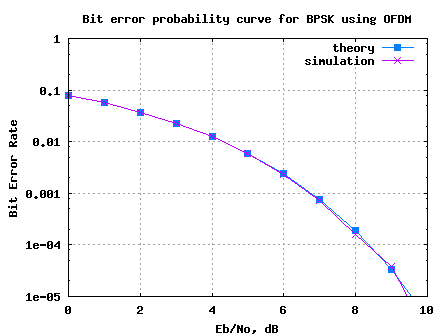- DSP log - http://www.dsplog.com -

BPSK BER with OFDM modulation

Posted By Krishna Sankar On June 10, 2008 @ 5:15 am In OFDM | 238 Comments

Oflate, I am getting frequent requests for bit error rate simulations using OFDM (Orthogonal Frequency Division Multiplexing) modulation. In this post, we will discuss a simple OFDM transmitter and receiver, find the relation between Eb/No (Bit to Noise ratio) and Es/No (Signal to Noise ratio) and compute the bit error rate with BPSK.

## OFDM modulation

Let us use the OFDM system loosely based on IEEE 802.11a specifications.

 Parameter Value FFT size. nFFT 64 Number of used subcarriers. nDSC 52 FFT Sampling frequency 20MHz Subcarrier spacing 312.5kHz Used subcarrier index {-26 to -1, +1 to +26} Cylcic prefix duration, Tcp 0.8us Data symbol duration, Td 3.2us Total Symbol duration, Ts 4us

You may refer to post Understanding an OFDM Transmission  for getting a better understanding of the above mentioned parameters.

## Cyclic prefix

In an OFDM transmission, we know that the transmission of cyclic prefix does not carry ‘extra’ information in Additive White Gaussian Noise channel. The signal energy is spread over time whereas the bit energy is spread over the time i.e.
.

Simplifying,

In OFDM transmission, all the available subcarriers from the DFT is not used for data transmission. Typically some subcarriers at the edge are left unused to ensure spectrum roll off. For the example scenario, out of the available bandwidth from -10MHz to +10MHz, only subcarriers from -8.1250MHz (-26/64*20MHz) to +8.1250MHz (+26/64*20MHz) are used.

This means that the signal energy is spread over a bandwidth of 16.250MHz, whereas noise is spread over bandwidth of 20MHz (-10MHz to +10MHz), i.e.

Simplifying,

.

## Relation between Eb/No and Es/No in OFDM

Combining the above two aspects, the relation between symbol energy and the bit energy is as follows:
.

Expressing in decibels,

.

## Simulation model

The attached Matlab/Octave simulation script performs the following:

(a) Generation of random binary sequence

(b) BPSK modulation i.e bit 0 represented as -1 and bit 1 represented as +1

(c) Assigning to multiple OFDM symbols where data subcarriers from -26 to -1 and +1 to +26 are used, adding cyclic prefix, concatenation of multiple symbols to form a long transmit sequence

(e) Grouping the received vector into multiple symbols, removing cyclic prefix, taking the desired subcarriers

(f) Demodulation and conversion to bits

(g) Counting the number of bit errorsFigure: Bit Error Rate plot for BPSK using OFDM modulation

Can observe that the simulated bit error rate is in good agreement with the theoretical bit error rate for BPSK modulation i.e.
.